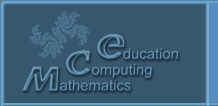Русский
 ! Вопросы и ответы

# Abstracts

## Current problems of mathematical reasoning science among schools` and universities` students

Pardala A.

Rzeszow University of Technology, The Faculty of Mathematics and Applied Physics, Department of Mathematics. 35-959 Rzeszów, Aleja Powstańców Warszawy 8; Poland. Phone: +48 17 865 1100 ; fax: +48 17 854 1260 ; E-mail: pardala@prz.edu.pl

2 pp. (accepted)

Problems of mathematical reasoning science among the schools’ and universities’ students are strictly related to contemporary researches conducted to improve and enhance the level of the quality of teaching mathematics. Previous work and results of creative mathematicians and mathematics educators shed light on some aspects of how to resolve these difficulties as well as specific learning difficulties of mathematical reasoning of these students, see:  – .

Contemporary Polish documents for mathematics education of universities’ students, the expected future mathematics teachers, and the third and fourth stage of school education students, see: , formulate and articulate the need of reaching the aims of the doctrine of mathematical reasoning. There are inter alia the following objectives: a) the ability to use and create information in mathematics, b) the ability to mathematical reasoning and argumentation, and the ability to gain and develop the related mathematical activity.

The lecture will be focused on the synthesis of theoretical knowledge, research questions and appropriately selected examples of research and teaching practice related to mathematical reasoning science of these students. Here are the research questions, which should be analysed and answered by mathematics teachers of universities and schools: 1) how to organize the analysis of students’ mathematical reasoning?, 2) how to correct the errors disclosed in students’ mathematical reasoning?, 3) how to organize creative mathematical activities aimed to discover the new mathematical concepts and their features as well as mathematical theorems?

References.

1. Ciosek M., Żeromska A.K. Rozumowanie w matematyce elementarnej, WN UP Kraków 2013, 92 s.

2. Гусев В.А. Теоретические основы обучения математике в средней школе: психология математического образования: учебное пособие для вузов, -М.: Дрофа, 2010, 473 с.

3. Krygowska A.Z. Zrozumieć błąd w matematyce, Dydaktyka Matem. 10(1989), s.141-147.

4. Pardała A. Kształtowanie twórczości w nauczaniu matematyki a praktyka szkolna i nauczycielska, Dydaktyka Matematyki 26(2004), s. 265 – 286.

5. Steinhaus H. Błędy w matematyce, Wiadomości Matematyczne XI(1969), s. 101- 108.

6. http://bip.men.gov.pl/men_bip/akty_prawne/rozporządzenie_20081233_v2.pdf .

© 2004 Designed by Lyceum of Informational Technologies №1533